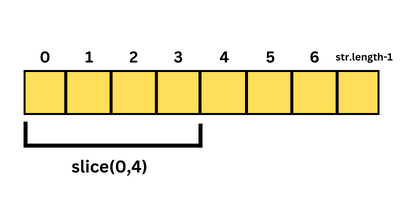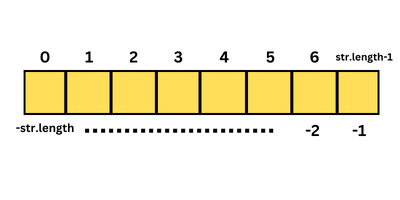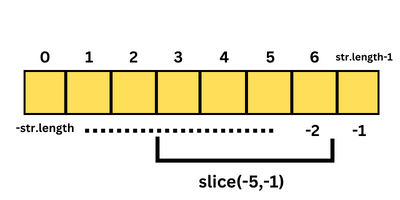# JavaScript String slice – a detailed look

In this tutorial, we’re going to get a detailed look into the JavaScript string slice method. We’re going to look at the basics of it, then how to use the various arguments at our disposal, how to send negative values as arguments, how the negative values change the output, and a lot more.

So, let’s get right into it then.

## JavaScript string slice method – an Introduction

As the name of the method implies, the ‘slice’ method is used to slice a portion of a string, to either extract it, or later remove it from the string.

For example, I can extract just the first 3 characters of the string ‘Hello’ and I’ll get back ‘Hel’. Now, I can reassign this sliced value to the original string to change it, or to a new variable if I want to keep the original string intact.

Or I can use another pre-defined string method in JavaScript like maybe the ‘replace’ method to replace the extracted string with an empty string, and effectively remove it from the original string.

These are just some of the use cases of the slice method. As you start programming in real-world environments, you’ll come up with a lot more.

### Slice method syntax

Now that we know how the string slice method works, let’s look at its syntax.

`string.slice(starting_pos, ending_pos);`

As you can see above, the slice method is preceded by the string’s variable name, which is ‘string’ in the above syntax. It also takes 2 arguments, the first one being the starting position and the second one being the ending position.

I’ll explain what the arguments entail in the next section.

### Slice method explained – with illustration

The slice method slices the string from the starting position to ending_pos – 1 (the character before the character at ending_pos). It doesn’t include the character at the ending_pos while slicing.

Let’s take the below as our example string.

`let str = 'This is my string';`

Now, let’s say I want to slice the first 4 characters, which amount to ‘This’ out of the string. I can obviously use the ‘slice’ method.

As you know, string indices start from 0, and go till string.length – 1, so I can give the starting position as 0 for my example, and the ending position as 4.

Why 4, and not 3? Well, the slice method only slices starting_pos to ending_pos – 1, which means it leaves out the character pertaining to the actual ending_pos index, so to include ‘s’, which is at the 3rd index in our string, we need to give the ending position as 4 and not 3, as shown in the image below:### Slice in action – Examples

So, now that we know how everything works in theory, let’s look at the final code snippet to achieve our desired result:

```let str = 'This is my string';
str = str.slice(0,4)
console.log(str); //'This' ```

There you go!

The slice() method as such doesn’t affect the original string. The sliced section is a copy that can be reassigned to the original variable, or to a new variable, as shown below:

```let str = 'This is my string';
let slicedWord = str.slice(0,4)
console.log(str); //'This is my string'
console.log(slicedWord); //'This' ```

As you can see, the slice() method’s result was placed in slicedWord, and it didn’t affect ‘str’, the original string, at all.

### Optional argument

The second argument is optional and leaving it out will extract the string from the starting position to the end of the string.

``` let str = 'This is my string';
str = str.slice(4);
console.log(str); //'is my string' ```

As you can see above, we’ve asked the slice() method to extract the string from the 4th position till the end of the string. Since spaces also take up an index in strings, the 4th position, which is occupied by the first space after ‘This’ will also be omitted and ‘is my string’ will be returned as the result.

## No or invalid arguments to the string.slice() method

If you give no arguments with the slice() method, it’ll just extract the entire string by default, as seen below:

```str = str.slice();
console.log(str); //'This is my string' ```

Also, if you give invalid arguments (NAN or undefined) as the first argument, and leave the second argument empty, you’ll get the entire string by default, just like the previous example.

``` str = str.slice('a');
console.log(str); //'This is my string' ```

As you can see, the result is the same for ‘undefined’:

``` str = str.slice(undefined);
console.log(str); //'This is my string' ```

But, after the NaN or undefined first argument, if you give a second valid argument, it’ll consider the first argument as 0 by default, and proceed as usual.

In the example below, the first argument is a NaN value (a character), but the second argument is 4, so your browser will assume that you want to extract from 0 to 4-1 (3), which is ‘This’.

```let str = 'This is my string';
str = str.slice('a',4);
console.log(str); //'This' ```

Similarly, if second argument is undefined, but the first argument is valid, it will extract from where the first argument starts in the string to the end of the string.

In the example below, the first argument is 5 (which means ‘This ‘ will be removed) and the second argument is undefined, so it’ll extract from the 5th position till the end of the string, which would be ‘is my string’.

```let str = 'This is my string';
str = str.slice(5,undefined);
console.log(str); //'is my string' ```

Please note that the final example will not work when the second argument is a NaN value. In that case, it’ll extract nothing, and you’ll end up with an empty string, as shown below:

```str = str.slice(5,NaN);
console.log(str); //'' ```

## More misc argument types

If the ending position (second argument) is lesser than the starting position (first argument), which wouldn’t make any logical sense for your browser, you’ll get back an empty string, as shown below:

``` str = str.slice(10,5);
console.log(str); //'' ```

On the other hand, if the ending position is greater than the string’s length, your browser will just extract until the end of the string and leave it at that.

```let str = 'This is my string';
console.log(str.length); //17
str = str.slice(5,20);
console.log(str); //'This is my string' ```

As you can see above, the length of the string is 17, but we’ve asked the browser to slice the string from positions 5 to 20, where 20 is greater than the string length. It doesn’t matter though. Our browser still extracted only until the end of the string with no issues.

## Negative values

Alternatively, you can also give negative values as your first and/or second arguments. These values work a little differently than their positive counterparts though.

For instance, giving -1 as the first argument, while leaving the second argument empty, will extract the last character in the string, as shown below:

```str = str.slice(-1);
console.log(str); //'g' - last character of the string ```

Now, this might be a little confusing, so let me explain things a bit. Just like how the positive indices of a string start from 0 and end at string.length-1, the negative indices start from -1, but the counting starts from the end of the string.

Look at the picture below:As you can see, the last character of the string has an index of -1, the second last character has an index of -2 and so on, while the first character of the string has an index of -str.length.

Now, this is a little different from the positive indices where the first character is at 0 and the last character is at str.length-1 (and not str.length), since the first character started at 0, and not 1.

So, if you’d like to slice just the last character of the string (extract the last character), give only -1 as your argument, which will extract the character at -1, and that’s it, as shown in the example above.

Also, you can slice from a positive starting position to a negative ending position. For instance, slicing from 5 to -1 will give us the substring at 5 to str.length – 2, as shown below:

```str = str.slice(5,-1);
console.log(str); //'is my strin' - 5 to str.length-2 ```

Now, make sure that the character at the ending position, even if it’s a negative number is not before the character at the starting position. If it is, as you know, the arguments won’t make any logical sense to your browser, and you’ll end up with an empty string, as shown below:

```let str = 'Hello';
str = str.slice(3,-4);
console.log(str); //'' ```

As you can see above, at the starting position 3, we have the character ‘l’ (the second ‘l’ in Hello), and at the ending position -4, we have ‘e’, which is before ‘l’ in the string ‘Hello’. Now that doesn’t make any logical sense, so we’ve gotten back an empty string.

Similarly, while giving a negative value as the starting position, and a positive value as the ending position, you’ll end up with an empty string depending on where the characters at each position are.

For example, in the example below, we’re trying to slice from the last character to the character at the 3rd index, which is just not possible, so we’ve ended up with an empty string.

```str = str.slice(-1,2);
console.log(str); //'' - empty string - not a valid set of arguments ```

On the other hand, in the example below, we’re trying to slice from -4 to 4-1 (3), where at -4, we have ‘e’, and at 3, we have the second ‘l’ in ‘Hello’, as shown below, so we’ll get back ‘ell’ as a result of the slice operation.

```let str = 'Hello';
str = str.slice(-4,4);
console.log(str); //'ell' ```

You can give a negative value as your starting position (first argument) and nothing as your ending position (second argument), as shown below:

```let str = 'Hello';
str = str.slice(-4);
console.log(str); //'ello' ```

As you can see, at -4, we have ‘e’, so our program slices from ‘e’ to the end of the string, which is ‘ello’.

Also, you can use 2 negative values as arguments for your slice() method. Just make sure that the first argument has a bigger negative number than the second argument, so you slice something other than an empty string, as shown in the illustration below:As shown in the example below, at -4, we have the character ‘e’, and at -2-1 (-3), we have ‘l’, so after processing, we end up with ‘el’.

```let str = 'Hello';
str = str.slice(-4,-2);
console.log(str); //'el' ```

There you go! We’ve looked at the different ways of using the JavaScript string slice method to slice different pieces of a given string.